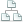Home >Tips >Excel sales calculationSite map

# Use Formulas in Excel, Sales Calculation

## An Excel tutorial by Peter KalmstromThe possibility to tell Excel to calculate is a crucial feature and the most important reason to use it. In two earlier demos Peter Kalmstrom has showed how to create Excel formulas for plus and minus and how to make Excel multiply and divide.

In the demo below Peter shows how to use these formulas in a more complicated calculation. As an example he uses a sales calculation where the input values are:
• VAT, in percent
• Margin, in percent
• Product in-price
• Number of sold items
• Discounts
From this Peter calculates:
• VAT, in dollars
• Margin, in dollars
• Product out-price
• Price to customer
• Total sales sum

Peter uses Excel 2013 for his demo, but the formulas are the same for other versions of Excel.

Follow kalmstrom.com on LinkedIn Facebook or Twitter!

 Products Buy FAQ Services Tips Books Contact About Us Tools
 Security and integrity Copyright  Kalmstrom Enterprises AB  All rights reserved